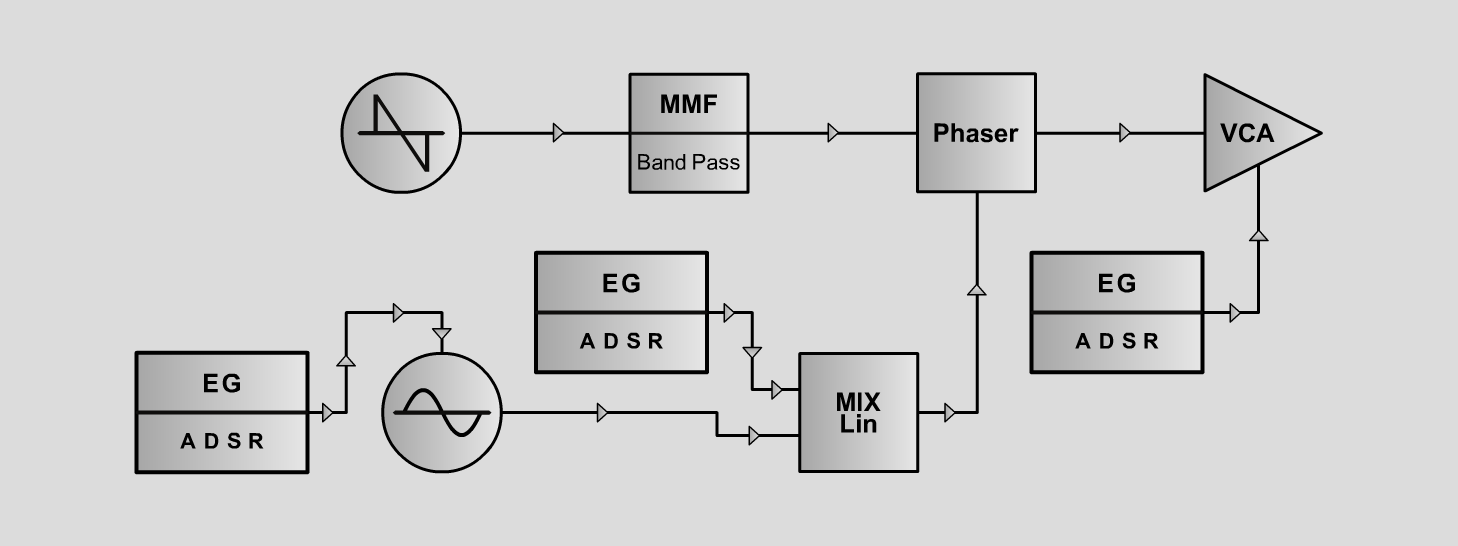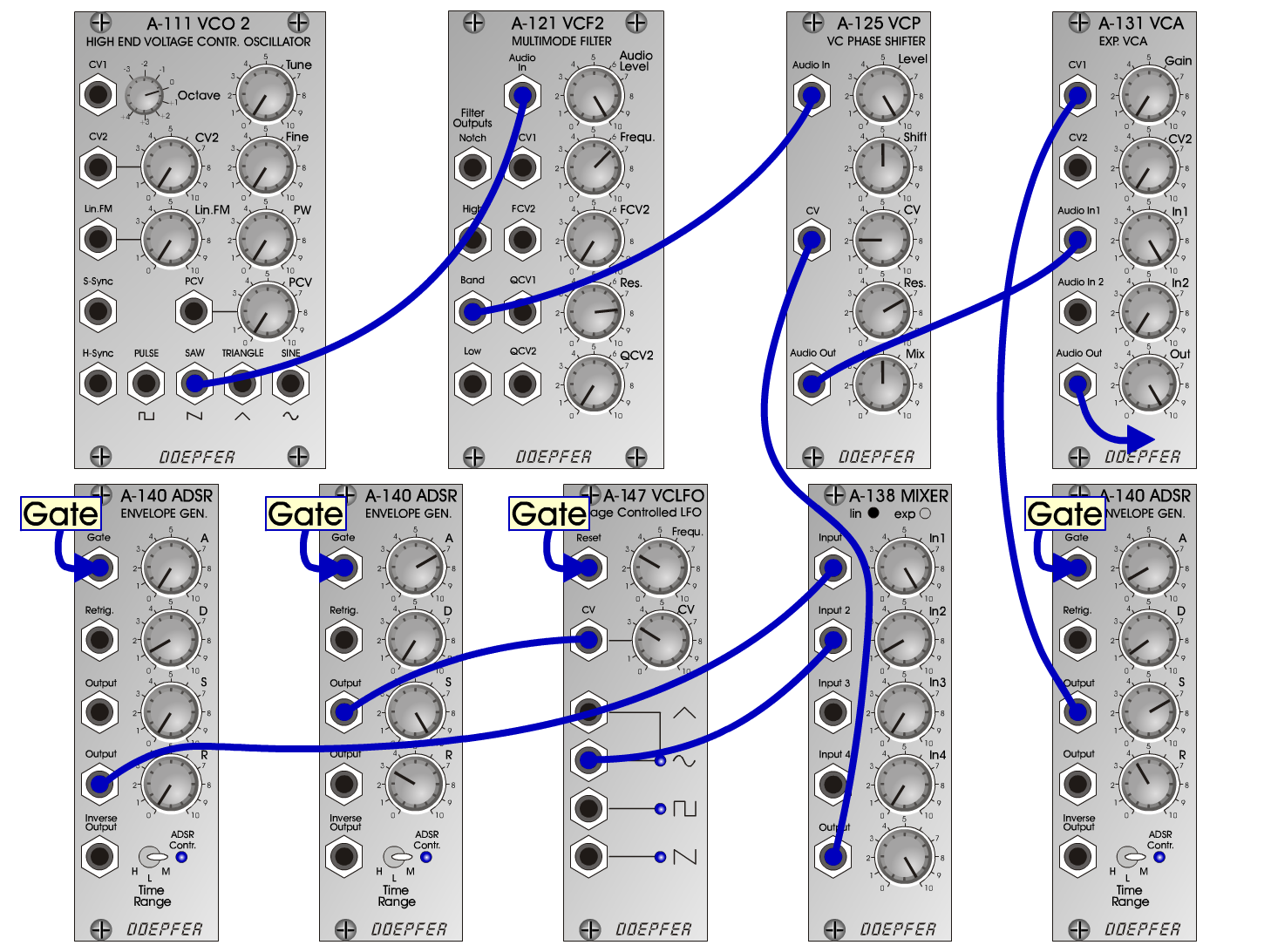O b o eInstrumentsD o e p f e r A - 100 Connections: Settings: Oboe 1: A-111 (Saw)  <=> A-121 (Audio In)   A-121 (Band) <=> A-125 (Audio In)   A-125 (Audio Out) <=> A-131 (Audio In)   A-138Lin (Output) <=> A-125 (CV)   A-140/1 (Output) <=> A-138Lin (Input 1)   A-121 (Low) <=>  A-131 (Audio In 1)   A-140/2 (Output) <=> A-131 (CV1)   A-140/3 (Output) <=> A-147 (CV)   A-147 (Sine) <=> A-138 (Input 2) A-121 (Audio Level = 10, Freq = 6.5, Res = 7.8)   A-125 (Level = 10, Shift = 5, CV = 2, Res = 7, Mix = 5)   A-131 (Gain = 0, Audio In 1 = 10, Audio Out = 10)   A-138 (In 1 = 10, In 2 = 1, Out = 10)   A-147 (Freq = 3, CV = 3)   A-140/1 (A = 0, D = 1, S = 0, R = 0, Range = M)   A-140/2 (A = 1, D = 0.8, S = 7, R = 4, Range = M)   A-140/3 (A = 7, D = 0, S = 10, R = 3, Range = M) Oboe 2: A-138 (In 1 = 10, In 2 = 0, Out = 10) Oboe 3: A-138 (In 1 = 0, In 2 = 0, Out = 0)   A-140/2 (A = 1.5, D = 0.8, S = 7, R = 4, Range = M) Oboe 4: A-138 (In 1 = 0, In 2 = 0.5, Out = 10)   A-140/2 (A = 1.5, D = 0.8, S = 7, R = 4, Range = M) Josef MuellerSound samples Bach: Kantate, Oboe I + II (BWV 8)     (Patch: Oboe3) Bach: Kantate (BWV 8)     (Patches: Oboe3, Strings, Clarinette, Solar-Flute) Oboe 1 A Oboe 1 B Oboe 1 C Oboe 2 A Oboe 2 B Oboe 2 C Oboe 3 A Oboe 3 B Oboe 3 C